CBSE Class 10 Sample Paper for 2020 Boards - Maths Standard

Class 10
Solutions of Sample Papers for Class 10 Boards

## Question 40 Daily wages of 110 workers, obtained in a survey, are tabulated below: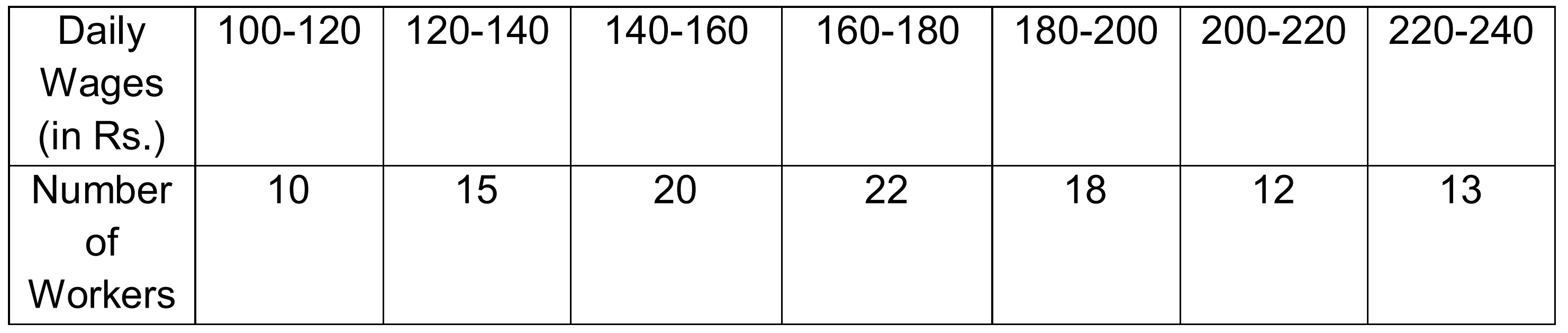(adsbygoogle = window.adsbygoogle || []).push({}); Compute the mean daily wages and modal daily wages of these workers.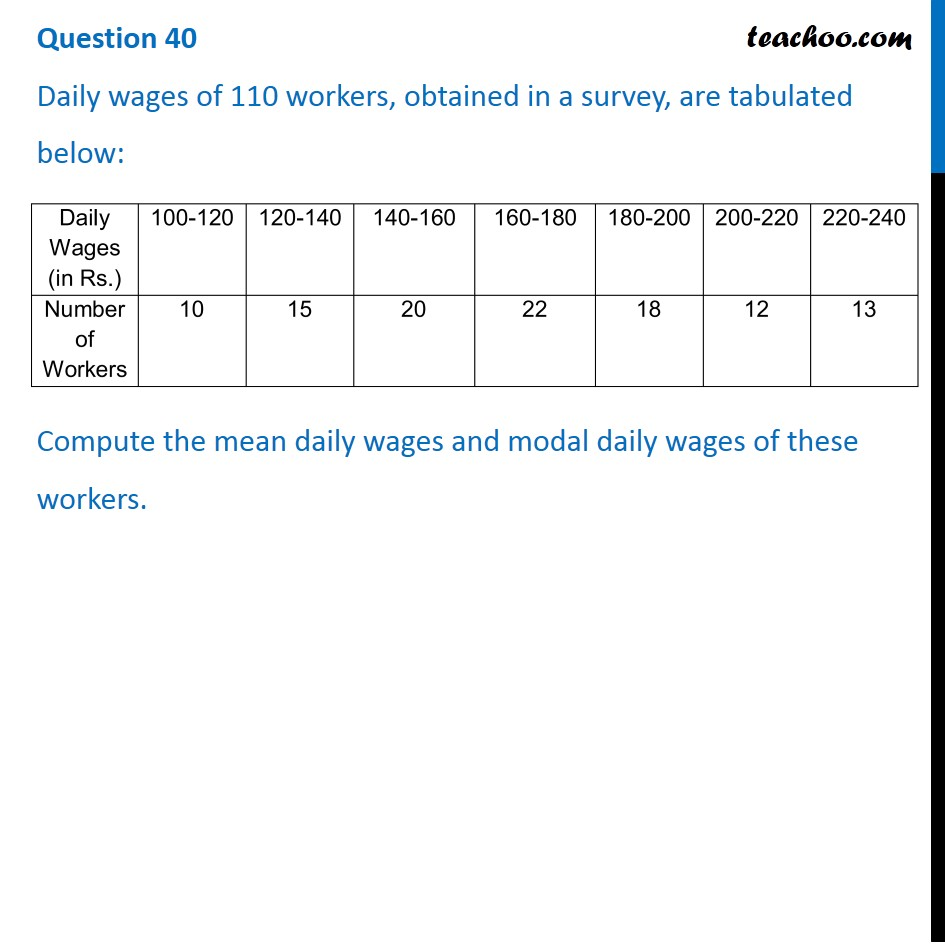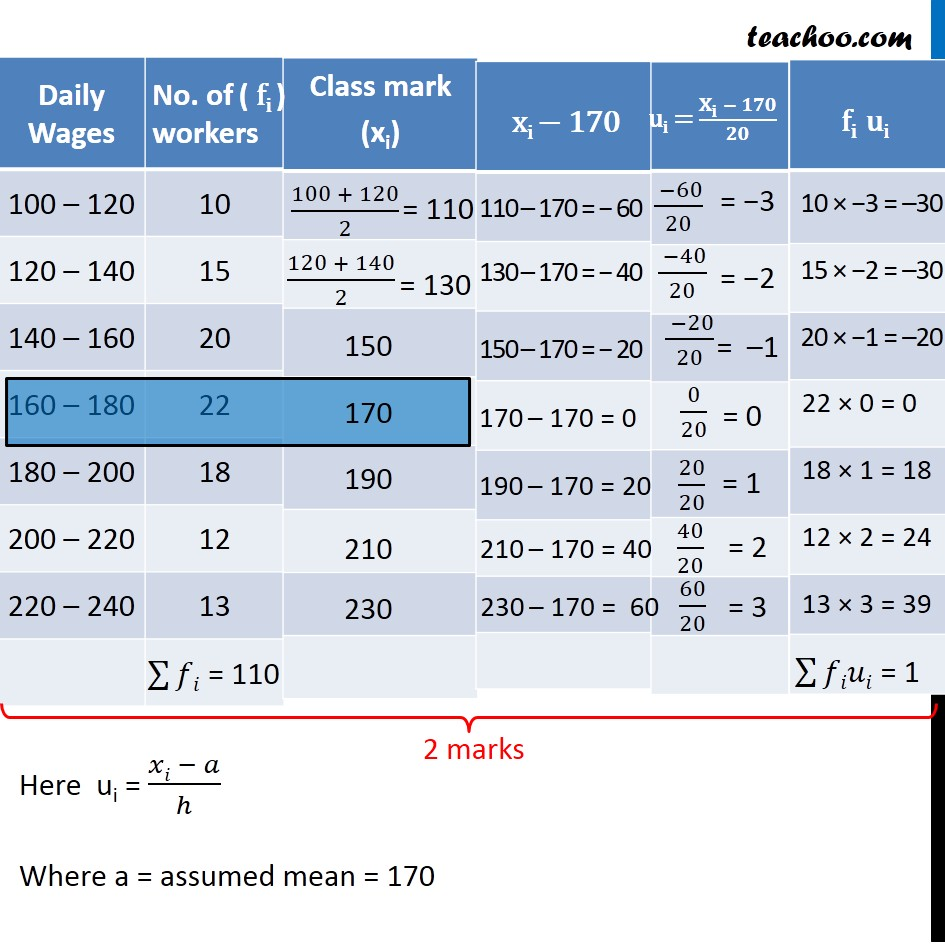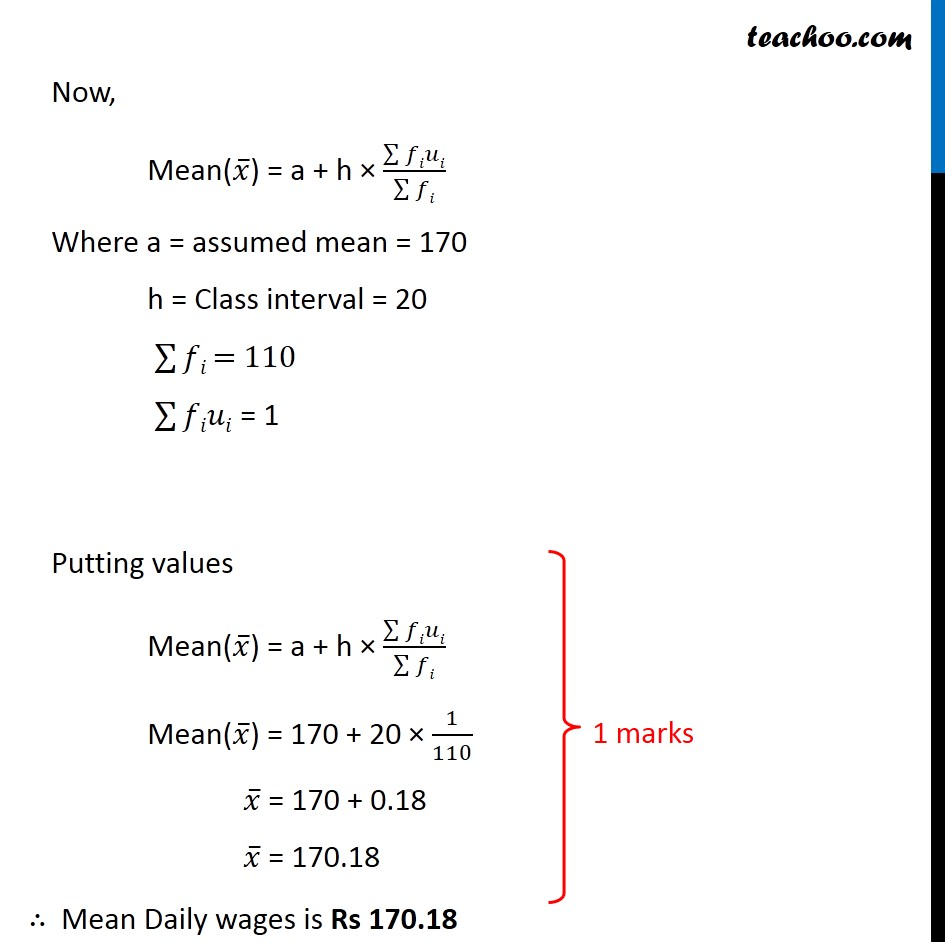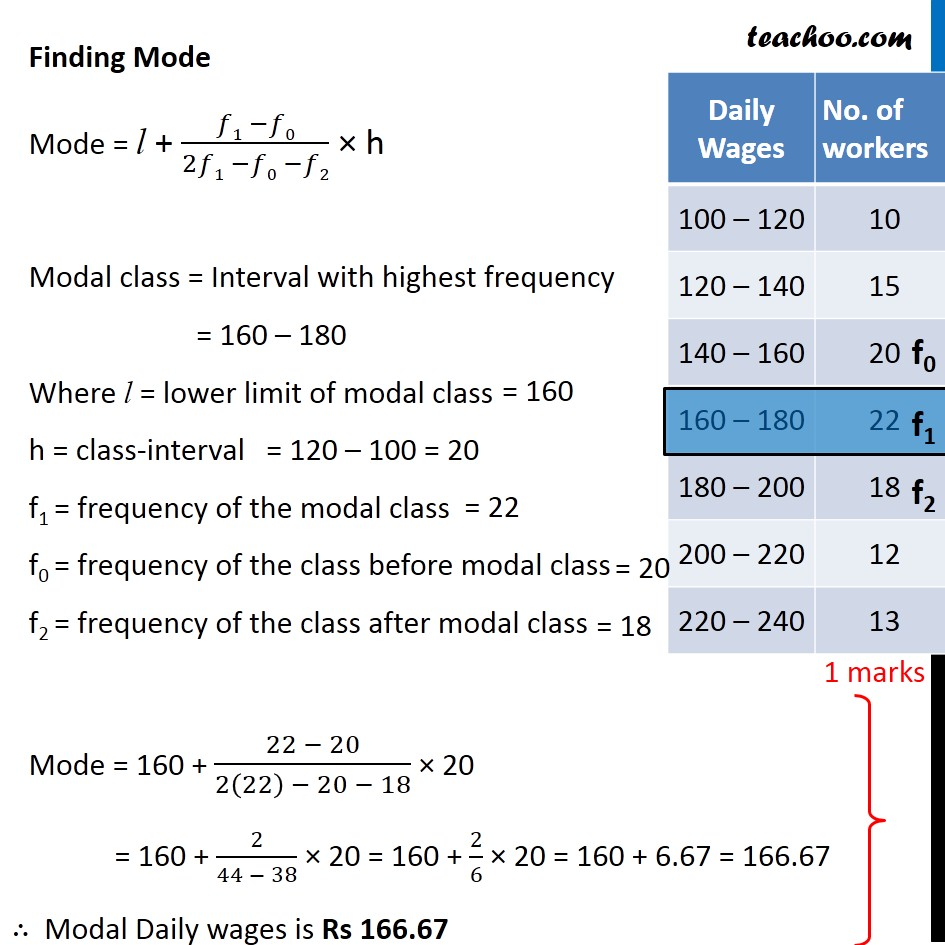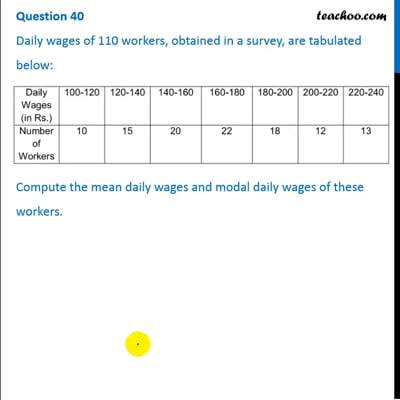This video is only available for Teachoo black users

### Transcript

Question 40 Daily wages of 110 workers, obtained in a survey, are tabulated below: Compute the mean daily wages and modal daily wages of these workers. Daily Wages No. of workers 100 – 120 10 120 – 140 15 140 – 160 20 160 – 180 22 180 – 200 18 200 – 220 12 220 – 240 13 Here ui = (𝑥𝑖 − 𝑎)/ℎ Where a = assumed mean = 170 Now, Mean(𝑥 ̅) = a + h × (∑▒𝑓𝑖𝑢𝑖)/(∑▒𝑓𝑖) Where a = assumed mean = 170 h = Class interval = 20 ∑▒𝑓𝑖 = 110 ∑▒𝑓𝑖𝑢𝑖 = 1 Putting values Mean(𝑥 ̅) = a + h × (∑▒𝑓𝑖𝑢𝑖)/(∑▒𝑓𝑖) Mean(𝑥 ̅) = 170 + 20 × 1/110 𝑥 ̅ = 170 + 0.18 𝑥 ̅ = 170.18 ∴ Mean Daily wages is Rs 170.18 Finding Mode Mode = l + (𝑓1 −𝑓0)/(2𝑓1 −𝑓0 −𝑓2) × h Modal class = Interval with highest frequency = 160 – 180 Where l = lower limit of modal class h = class-interval f1 = frequency of the modal class f0 = frequency of the class before modal class f2 = frequency of the class after modal class Mode = 160 + (22 − 20)/(2(22) − 20 − 18) × 20 = 160 + 2/(44 − 38) × 20 = 160 + 2/6 × 20 = 160 + 6.67 = 166.67 Daily Wages No. of workers 100 – 120 10 120 – 140 15 140 – 160 20 160 – 180 22 180 – 200 18 200 – 220 12 220 – 240 13 ∴ Modal Daily wages is Rs 166.67